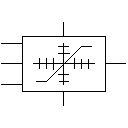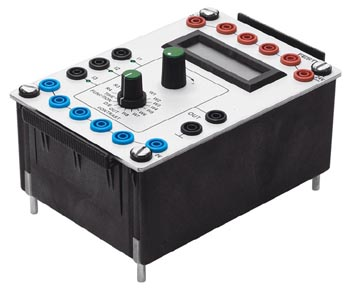# Setpoint value cardVoltage profiles in the range -10 V to +10 V can be created using the setpoint value card. Up to 8 setpoints W1 to W8 can be specified in the voltage range -10 V to +10 V. The setpoint card requires a power supply of 24 V.

The increase from the current setpoint to the next setpoint is defined using 4 ramps R1 to R4 with values between 0 s/V and 10 s/V, i.e. a low ramp value signifies a large increase, whereas a high ramp value results in a small increase. The active ramp is defined as follows: R1 by a positive increase of 0 V, R2 by a negative increase up to 0 V, R3 by a negative increase of 0 V and R4 by a positive increase up to 0 V.

Three operating modes can be selected: Wait for switching time, Advance setpoints and External control.

In operating mode Wait for switching time the setpoints are sequentially advanced when the preset change over time has expired.

If Advance setpoints is selected then, upon attaining the active setpoint, the next setpoint is started without delay.

In operating mode External control the selection of the active setpoint is effected by gating the inputs I1, I2 and I3 with at least 15 V. The corresponding setpoint is selected by means of the specified bit table. During the process, the internal switching time is inactive.

 W1: I1=0 I2=0 I3=0 W2: I1=1 I2=0 I3=0 W3: I1=0 I2=1 I3=0 W4: I1=1 I2=1 I3=0 W5: I1=0 I2=0 I3=1 W6: I1=1 I2=0 I3=1 W7: I1=0 I2=1 I3=1 W8: I1=1 I2=1 I3=1